# Change The Shape Of A Parabola (4 Shifts & Transformations)

Graphing a parabola is one thing, but making a change so that it has a different shape or position is another.  Changing the shape of a parabola involves adding and multiplying by certain numbers – but the trick is to know which numbers to use and when to use them.

So, how do you change the shape of a parabola?  You can shift a parabola horizontally or vertically by adding to x or y in the quadratic equation.  You can change the width by multiplying the quadratic equation by a nonzero number.  You can change the concavity by multiplying the quadratic equation by a negative number (or make a < 0).

Of course, it is important to know exactly how each of these changes will affect the appearance of a parabola on a graph.

In this article, we’ll take a look at the four basic changes you can make to the graph of a parabola.  We’ll also look at some examples to make the concepts clear.

Let’s get started.

## How To Change The Shape Of A Parabola

You can change the appearance of a parabola in 4 basic ways.  These shifts and transformations (or translations) can move the parabola or change how it looks:

• Horizontal Shift – this moves the entire parabola left or right without changing its basic shape.
• Vertical Shift – this moves the entire parabola up or down without changing its basic shape.
• Width Change – this makes the parabola wider or narrower, changing its basic shape.
• Negative Reflection (concavity change) – this reflects the parabola across the x axis, changing its concavity.

Let’s take a closer look at each of these changes and how they work.  We’ll start with the horizontal shift.

### How To Move A Parabola To The Right Or Left (Horizontal Shift)

To move a parabola H units to the right, you would substitute x – H for x in the corresponding quadratic equation.  This is called a horizontal shift.

For example, if we have the quadratic function f(x) = x2, then we would plug in x – S to get f(x – H) = (x – H)2.  If you wanted to FOIL and combine like terms, the new quadratic would be f(x – H) = x2 – 2Hx + H2.

This second parabola f(x – H) has the same shape as the original parabola f(x), but it is shifted H units to the right.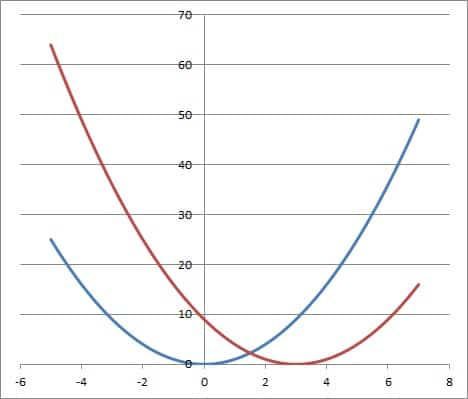The graph of the quadratic function f(x) = x2 (blue curve) and f(x – 3) (red curve), which is shifted 3 units to the right (H = +3).

Here are the 3 possible cases for H (in terms of sign and the resulting horizontal shift):

• H > 0: in this case, the graph of f(x – S) will be the graph of f(x) shifted H units to the right.
• H= 0: in this case, the graph of f(x – S) will be the same as the graph of f(x) (that is, no shift).
• H < 0: in this case, the graph of f(x – S) will be the graph of f(x) shifted |H| units to the left.

Let’s look at examples of both right and left horizontal shifts.

#### Example 1: Horizontal Shift To The Right

Let’s say we have the parabola f(x) = x2 + 6x + 8.  If we want to shift the parabola 4 units to the right, then we have H = +4.

To find the equation of the shifted parabola, we substitute x – H for x in the original parabola equation:

• f(x) = x2 + 6x + 8  [original quadratic equation corresponding to the parabola]
• f(x – 4) = (x – 4)2 + 6(x – 4) + 8  [substitute x – 4 for x]
• f(x – 4) = x2 – 8x + 16 + 6x – 24 + 8  [FOIL (x – 4)2 and distribute 6 to parentheses]
• f(x – 4) = x2 – 2x  [combine like terms]

So, the quadratic f(x – 4) = x2 – 2x is a parabola with the same shape as f(x) = x2 + 6x + 8, but shifted 4 units to the right.The quadratic function f(x) = x2 + 6x + 8 (blue curve) and f(x – 4) (red curve), which is shifted 4 units to the right (H = +4).

#### Example 2: Horizontal Shift To The Left

Let’s say we have the parabola f(x) = x2 + 8x + 15.  If we want to shift the parabola 2 units to the left, then we have H = –2

To find the equation of the shifted parabola, we substitute x – H for x in the original parabola equation (note that x – H = x – (-2) = x + 2):

• f(x) = x2 + 8x + 15  [original quadratic equation corresponding to the parabola]
• f(x + 2) = (x + 2)2 + 8(x + 2) + 15  [substitute x + 2 for x]
• f(x + 2) = x2 + 4x + 4 + 8x + 16 + 15  [FOIL (x + 2)2 and distribute 8 to parentheses]
• f(x – 4) = x2 + 12x + 35  [combine like terms]

So, the quadratic f(x + 2) = x2 + 12x + 35 is a parabola with the same shape as f(x) = x2 + 8x + 15, but shifted 2 units to the left.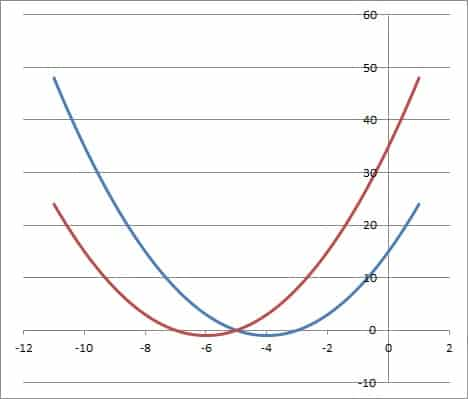The quadratic function f(x) = x2 + 8x + 15 (blue curve) and f(x + 2) (red curve) shifted 2 units to the left (H = -2).

### How To Move A Parabola Up Or Down (Vertical Shift)

To move a parabola V units up, you would substitute y – H for Y in the corresponding quadratic equation.  This is called a vertical shift.

For example, if we have the quadratic y = x2, then we would substitute y – S for y to get y – V = x 2.  If you wanted to solve for y, the new quadratic would be y = x2 + V.

This second parabola y = x2 + V has the same shape as the original parabola y = x2, but it is shifted V units up.

Here are the 3 possible cases for V (in terms of sign and the resulting vertical shift):

• V > 0: in this case, the graph of y + V will be the graph of y shifted V units up.
• V= 0: in this case, the graph of y + V will be the same as the graph of y (that is, no shift).
• V < 0: in this case, the graph of y + V will be the graph of y shifted |V| units down.

If V is not zero, the two parabolas will never intersect. If we set them equal, we will get an equation with no solution.

Let’s look at examples of both up and down vertical shifts.

#### Example 1: Vertical Shift Up

Let’s say we have the parabola y = x2 + 6x + 8.  If we want to shift the parabola 3 units up, then we have V = +3.

To find the equation of the shifted parabola, we substitute y – V for y in the original parabola equation:

• y = x2 + 6x + 8  [original quadratic equation corresponding to the parabola]
• y – 3 = x2 + 6x + 8  [substitute y – 3 for y]
• y = x2 + 6x + 8 + 3  [add 3 to both sides to solve for y]
• y = x2 + 6x + 11  [combine like terms]

So, the quadratic y = x2 + 6x + 11 is a parabola with the same shape as y = x2 + 6x + 8, but shifted 3 units up.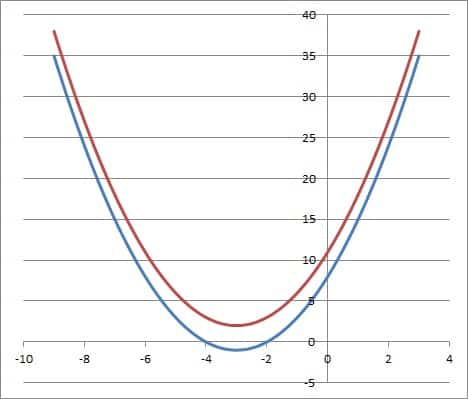The graph of the quadratic function y = x2 + 6x + 8 (blue curve) and y + 3 (red curve), shifted up 3 units.

#### Example 2: Vertical Shift Down

Let’s say we have the parabola y = x2 + 6x + 5.  If we want to shift the parabola 4 units down, then we have V = -4.

To find the equation of the shifted parabola, we substitute y – V for y in the original parabola equation (note that y – V = y – (-4) = y + 4):

• y = x2 + 6x + 5  [original quadratic equation corresponding to the parabola]
• y + 4 = x2 + 6x + 5  [substitute y + 4 for y]
• y = x2 + 6x + 5 – 4  [subtract 4 from both sides to solve for y]
• y = x2 + 6x + 1  [combine like terms]

So, the quadratic y = x2 + 6x + 1 is a parabola with the same shape as y = x2 + 6x + 5, but shifted 4 units down.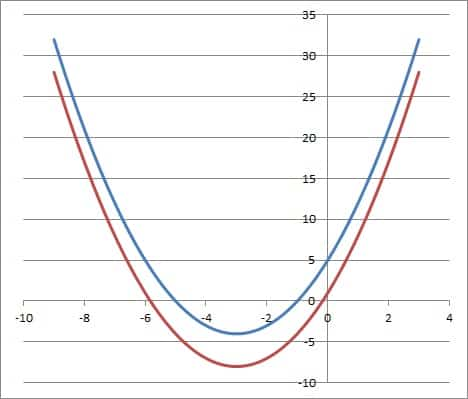The graph of the quadratic function y = x2 + 6x + 5 (blue curve) and y – 4 (red curve), shifted down 4 units.

### How To Make A Parabola Wider Or Narrower (Width Change)

To make a parabola wider, make the value of a (or |a|) larger.

Another way to make a parabola wider or narrower is to substitute Wx for x in the corresponding quadratic equation.  This is called a width change (it may also change the location of the parabola’s vertex).

For example, if we have the quadratic f(x) = x2, then we would plug in Wx for x to get f(Wx) = W2x2.

This second parabola f(Wx) has the a different shape and vertex than the original parabola f(x).

Here are the 3 possible cases for W (in terms of sign and the resulting width change):

• W > 1: in this case, the graph of f(Wx) will be the graph of f(x), but 1 / W2 as wide (that is, narrower than the original parabola).
• W = 1: in this case, the graph of f(Ws) will be the same as the graph of f(x) (that is, same width).
• 0 < W < 1: in this case, the graph of f(Wx) will be the graph of f(x), but 1 / W2 times as wide (that is, wider than the original parabola).

Let’s look at examples of both widening and narrowing width changes.

#### Example 1: Decreasing Width (W > 1)

Let’s say we have the parabola y = x2 + 6x + 8.  Let’s try a width change with W = +4.

To find the equation of the shifted parabola, we substitute Wx for x in the original parabola equation:

• f(x) = x2 + 6x + 8  [original quadratic equation corresponding to the parabola]
• f(4x) = (4x)2 + 6(4x) + 8  [substitute 4x for x]
• f(4x) = 16x2 + 24x + 8  [simplify]

So, the quadratic y = 16x2 + 24x + 8 is a parabola with 1/4 the width of the parabola y = x2 + 6x + 8.

#### Example 2: Decreasing Width (0 < W < 1)

Let’s say we have the parabola y = x2 + 8x + 16.  Let’s try a width change with W = 0.5.

To find the equation of the shifted parabola, we substitute Wx for x in the original parabola equation:

• f(x) = x2 + 8x + 16  [original quadratic equation corresponding to the parabola]
• f(0.5x) = (0.5x)2 + 8(0.5x) + 16  [substitute 0.5x for x]
• f(0.5x) = 0.25x2 + 4x + 16  [simplify]

So, the quadratic y = 0.25x2 + 4x + 16  is a parabola with 2 times the width of the parabola y = x2 + 8x + 16.

### How To Make A Parabola Upside Down (Negative Reflection Or Concavity Change)

Remember that a quadratic in standard form has the equation f(x) = ax2 + bx + c.  The coefficient of x2, a, will determine the concavity of the parabola (whether it opens up or down):

• A parabola will open upwards (concave up) if a > 0.
• A parabola will open downwards (concave down) if a < 0.

To make a parabola upside down (or change the concavity), simply multiply the quadratic equation by -1 on the right side.

This will reflect the parabola across the x axis.  A parabola that opened upward will now open downward, and vice versa.

For example, if we have the quadratic f(x) = x2, then we would multiply by -1 on the right side to get g(x) = -x2.

This second parabola g(x) = -x2 has the same shape than the original parabola f(x) = x2, but it opens downward, and it is reflected across the x axis.

Let’s look at an example of turning a parabola upside down.

#### Example: Turning A Parabola Upside Down

Let’s say we have the parabola y = x2 + 8x + 16.  If we want to turn the parabola upside down, then we multiply by -1 on the right side.

To find the equation of the shifted parabola, we change the sign of every coefficient:

• f(x) = x2 + 8x + 16  [original quadratic equation corresponding to the parabola]
• g(x) = -x2 – 8x – 16  [change the sign of every coefficient]

So, the quadratic g(x) = -x2 – 8x – 16is the parabola f(x) = x2 + 8x + 16, but turned upside down. Note that they have the same width, however.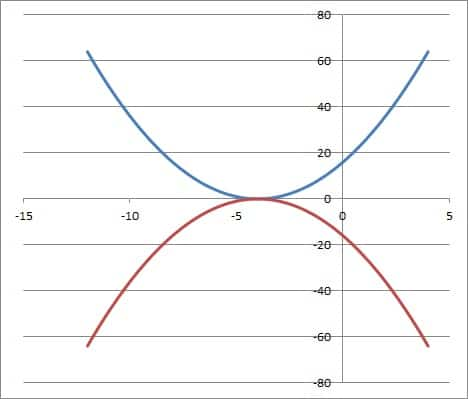The graph of the quadratic function f(x) = x2 + 8x + 16 (blue curve) and -f(x) = -x2 – 8x – 16 (red curve)

### Combining Multiple Shifts & Transformations

Of course, we can also combine multiple shifts and transformations with the same parabola.

For example, let’s start off with the parent function f(x) = x2, which is the most basic parabola we can think of.

First, we’ll shift f(x) left 2 units by substituting x + 2 for x.  This gives us (x+2)2 = x2 + 4x + 4.

So, g(x) = x2 + 4x + 4 is the original function f(x) shifted 2 units left.

Next, we’ll shift g(x) up by 3 units by subtracting 3 from y (or adding 3 to the right side).  This gives us x2 + 4x + 4 + 3 = x2 + 4x + 7.

So, h(x) = x2 + 4x + 7 is the original function f(x) shifted 2 units left and 3 units up.

Finally, we’ll turn i(x) upside down by changing the sign of all of the coefficients.  This gives us -4x2 – 8x – 7.

So, j(x) = -4x2 – 8x – 7 is the original function f(x) shifted 2 units left and 3 units up, with half the width and turned upside down.

## Conclusion

Now you know how to change the shape and position of a parabola by horizontal shifts, vertical shifts, and width changes.  You also know how to turn a parabola upside down.

You can learn how to find the domain and range of a parabola here.

You might also want to read my article on when quadratics are negative.

You might also want to read my article on common questions about ellipses (a parabola is a conic section, just like an ellipse).